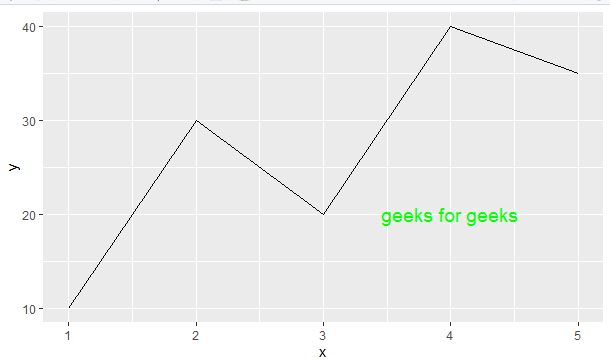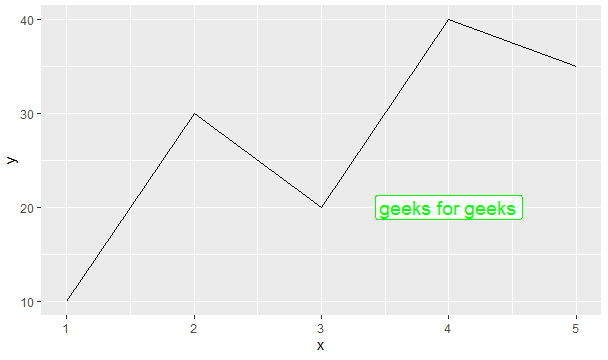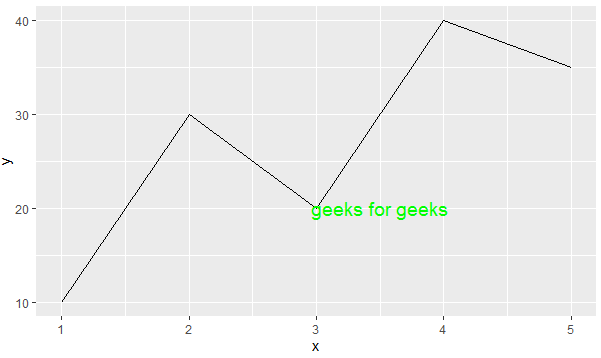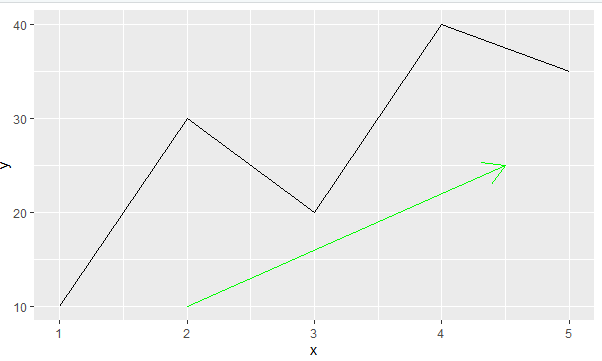How to annotate a plot in ggplot2 in R ?

• Last Updated : 29 Jun, 2021

In this article, we will discuss how to annotate plots in ggplot2 in R Programming Language.

An annotation can help the readability of a plot. It allows adding text to a plot or highlight a specific portion of the curve. The most common form of annotation is text. Let us first plot a regular plot without any annotation so that the difference is apparent.

Method 1: Using geom_text()

This allows annotating only text to a plot. This function along with the required parameters is added to the plot.

Syntax:

geom_text(data, x, y, label)

Parameter:

• data: dataframe in consideration
• x: x coordinate of text
• y: y coordinate of text
• label: text

To annotate using this function, first, a dataframe of the values is created and then the values to geom_title() are passed with reference to the dataframe so created.

Example:

R

 library("ggplot2")  x<-c(1, 2, 3, 4, 5)y<-c(10, 30, 20, 40, 35)  df<-data.frame(x, y)  ann_text<-data.frame(    x = 4, y = 20,    label = "geeks for geeks")  ggplot(df, aes(x,y))+geom_line()+geom_text(data = ann_text,           aes( x=x, y=y, label=label),          color="green", size=5)

Output:Method 2: Using geom_label()

This function is used to add labels i.e. text is framed in the plot, but since it does the job of adding an annotation this can be considered as one alternative.

Syntax:

geom_text(data, x, y, label)

Parameter:

• data: dataframe in consideration
• x: x coordinate of text
• y: y coordinate of text
• label: text

This method is similar to the above one, except the output produced for this will be treated as a label instead of a regular text, and will have a boundary around it.

Example:

R

 library("ggplot2")  x<-c(1, 2, 3, 4, 5)y<-c(10, 30, 20, 40, 35)  df<-data.frame(x, y)  ann_text<-data.frame(    x = 4, y = 20,    label="geeks for geeks")  ggplot(df,aes(x,y))+geom_line()+geom_label(data = ann_text,           aes( x = x, y = y, label=label),           color="green", size=5)

Output:Method 3: Using annotate()

annotate() function is most the commonly used function to add annotations to a plot. This not only allows text but also shapes to be displayed on the plot.

Syntax:

annotate(type, x, y,)

Parameter:

• type: type of annotation
• x: x coordinate
• y: y coordinate

To annotate a text to the plot pass the “text” as type and in the label argument, pass the text to be annotated.

Example:

R

 library("ggplot2")  x<-c(1, 2, 3, 4, 5)y<-c(10, 30, 20, 40, 35)  df<-data.frame(x,y)    ggplot(df,aes(x,y))+geom_line()+annotate(  "text", x=3.5, y=20, label="geeks for geeks",  color="green", size=5)

Output:To annotate a shape to the plot, the type argument is passed with the required type and then coordinates are set accordingly.

Example:

R

 library("ggplot2")  x<-c(1,2,3,4,5)y<-c(10,30,20,40,35)  df<-data.frame(x,y)    ggplot(df,aes(x,y))+geom_line()+annotate(  "segment", x=2, xend=4.5, y=10,yend=25,   color="green", arrow=arrow())

Output:My Personal Notes arrow_drop_up